## How to Calculate Sum or Count Based on Cell Colors

In this article we will do a step by step guide on how to add up values based on cell colors. And then we will go through how to count the number of cells that are of a particular color. We will be doing so using Visual Basic for Applications (VBA). However this method is not without its limitation. Before we end this article, we will explain what the limitation is and provide tips on how to work around it.

Before we start, as we mentioned, we will be using VBA to do this so please make sure VBA is enabled in your Excel file.

## Calculate SUM Based on Cell Colors

To calculate sum of a set of values based on colors of the cells, we will be creating very own user-defined function. This is something we have gone through in a previous article: User-Defined Functions – Writing Your Own Functions in Excel.

To begin:

1. Go to Developer tab
2. Click on Visual Basic
3. Click on Insert at the top
4. Select Module
5. Copy and paste the following code into the module:

The first step to writing any user-defined functions is always to always have a name for the function and also consider what variables/fields do we require from the users each time they use this function. In this case, we named the function SumByColor (you can call it something else) and there are two inputs we require each time:

1. The cell that has the color which we want to reference for this function: colorcell
2. The range of cells which the function will check if the cell color is colorcell (from above): cellrange

Now let’s explain the codes step by step:

1. First off, we need to let this function know the color it is looking for in the range of cells. And that is what the first variable or field is for: colorcell. Inside the module, we first create a variable: WhatColor – this variable stores the color index of the cell color for colorcell:

WhatColor = colorcell.Interior.Color
2. Next we create another variable called x – this variable will keep adding a new value onto itself if its cell color matches with WhatColor. At the start of this function, we will set:

x = 0

This is because at this point, we haven’t checked any cell in cellrange against WhatColor yet.
3. The next step is to run a loop. The loop will go through each cell in the cellrange specified in the function:

For Each coloredcell In cellrange

Note that the variable coloredcell can really be called anything. It’s just another variable we’re creating for this loop.
4. For each cell in the cellrange, there are two checks we want to do: 1) if the cell color matches WhatColor and 2) if the value in the cell is a number. If both are true, then we will add the value in that cell to x. And this new value becomes our new x:

If coloredcell.Interior.Color = WhatColor And IsNumeric(coloredcell) = True Then
x = x + coloredcell
End If

5. Once all the cells in cellrange is looped through, the final x will be the sum of all the values that are in cells of a particular color we want. And this is what we want the function to return:

SumByColor = x

Let’s test this out. We named our user-defined functions SumByColor so we should be able to see the function now if we enter it into a cell: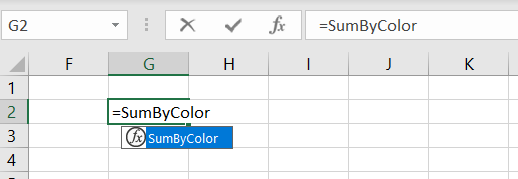There are two fields required: 1) colorcell and 2) cellrange: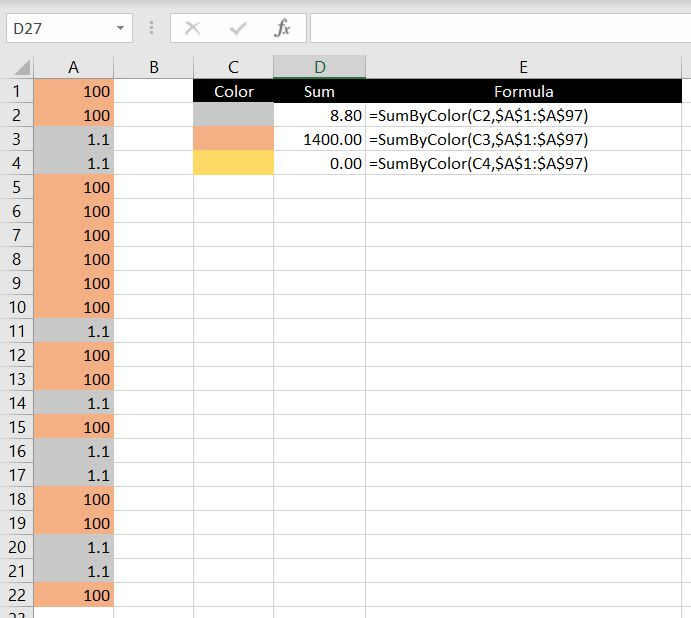=SumByColor(C2,\$A\$1:\$A\$97)

For each formula, we first reference the cell on the left (in the first formula: C2). This tells the function what color we are looking for. Not that we need to know but Excel looks at the color of this cell and stores the color index as WhatColor. And then Excel loops through each cell in range A1:A97. For each cell which has color matching WhatColor, the value gets added onto a variable called x. And at the end of the loop, the function returns the value of x.

Note that the “If IsNumeric(coloredcell) = True” is important because otherwise we could be asking Excel to do an addition with non-numerical values, in which case Excel would return #VALUE. But with this condition added to the IF statement, if Excel recognizes that the value is not numerical (even if the color matches), it will skip over this value: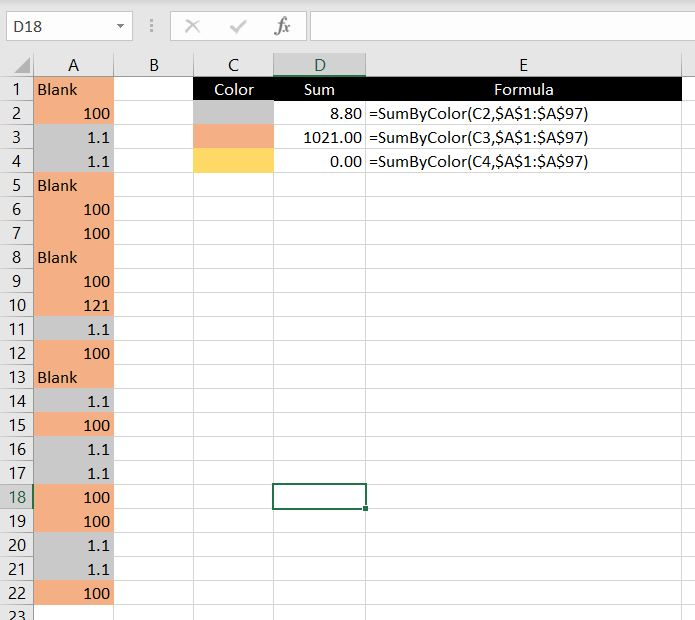## COUNT Number of Cells With A Particular Color

Next we will look at how to create a user-defined function that can count the number of cells that are filled with a particular color. Again, first thing to do would be to make sure our Excel file is macro-enabled.

Secondly we will need to think of a name for our function. In this case, we will call our function: CountByColor. Essentially you can give your function whatever name you want (except one that is already used by a built-in function in Excel) but it is good practice to choose a name that actually makes sense to you and to everyone who will be using your Excel file.

Similar to calculating the sum of values based on the cells’ colors, we need the exact same variables/inputs for this function:

1. The cell that has the color which we want to reference for this function: colorcell
2. The range of cells which the function will check if the cell color is colorcell (from above): cellrange

Now let’s explain the codes step by step:

1. First off, we need to let this function know the color it is looking for in the range of cells. And that is what the first variable or field is for: colorcell. Whatever color this cell is filled with, this function will match every cell in the second variable against this color. And we create a variable WhatColor to store this color index:

WhatColor = colorcell.Interior.Color
2. Next we create another variable called x – this variable will keep adding one to itself if the cell color matches with WhatColor. At the start of this function, we will set:

x = 0

This is because at this point, we haven’t checked any cell in cellrange against WhatColor yet.
3. The next step is to run a loop. The loop will go through each cell in the cellrange specified in the function:

For Each coloredcell In cellrange

Note that the variable coloredcell can really be called anything. It’s just another variable we’re creating for this loop.
4. For each cell in the cellrange, we want to check if the cell color matches WhatColor. If it does, then we will add one each time to x:

If coloredcell.Interior.Color = WhatColor Then
x = x + 1
End If

Optional: Note that in the SumByColor function, we had the additional check to make sure the cell value is numerical. We didn’t do that in this case since we are not adding any values together. But theoretically, we could add more conditions. E.g. we could make sure the cell is not blank by adding:

And Len(coloredcell)>0

In which case, the If statement becomes:

If coloredcell.Interior.Color = WhatColor And Len(coloredcell) > 0 Then
5. Once all the cells in cellrange is looped through, the final x will be the total number of cells that are filled with WhatColor. And this is what we want the function to return:

CountByColor = x

Let’s test this out. You should now be able to see a CountByColor function:There are two fields required: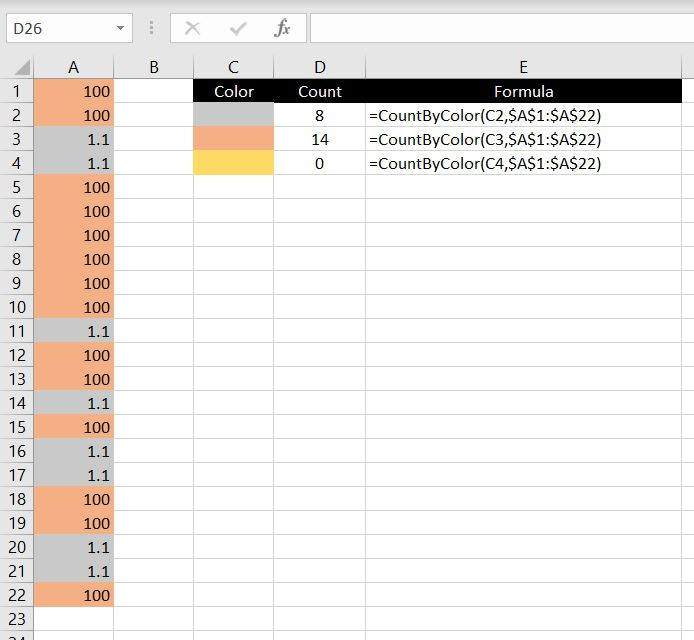We first reference the cell on the left (in the first formula: C2). This tells the function what color we are looking for. Excel then stores this color index as WhatColor and then it loops through each cell in range A1:A22. For each cell which has color matching WhatColor, the variable x adds one to itself. And at the end of the loop, the function returns the value of x.

As mentioned above, we could add more conditions depending on what we need. For example besides from the color of the cell, we could also test whether the value is numerical, or we could test whether the value is over 1000.

## What To Be Mindful Of…

Before we end the article, let’s go through what we need to be mindful of with these two user-defined functions above because there are key two limitations:

### 1) When Does The Function Recalculate Itself?

One thing to keep in mind is whether the function recalculates itself automatically and when. In Excel, if in a function you reference another cell or range of cells and let’s say the value in those referenced cell(s) changes, the value from your function will update itself automatically.

Rest assured this is the same with the user-defined functions we just created. However from Excel’s perspective, changing a background color of a cell is not the same as updating/changing a value in a cell. Simply put, changing a cell color is not a catalyst for Excel to recalculate its functions and formulas. This means if you add a number into a cell and then change the color of the cell, the two user-defined functions will not pick them up (at least not until a value is updated somewhere in the worksheet that will trigger Excel to recalculate all its functions and formulas).

#### Workaround:

Instead of entering a value into the cell and then changing the color of the cell, do it the other way around. You will need to first change the color of the cell and then update value in it. Simply put, it is the updating of the value that triggers Excel to recalculate all the functions and formulas. So by updating the value after changing the cell color, the user-defined functions will be updated.

### 2) This Does Not Work With Conditional Formatting

Unfortunately this just doesn’t work with conditional formatting. To illustrate this, we created another user-defined function: WhatColorCell. This function checks the color of a particular cell and returns the color index:

In the examples below, we used this function on three different cells 1) the first one is filled with red, 2) the second has conditional formatting such that if the cell value is over 200, it will be formatted as red and 3) the cell has no color filled at all: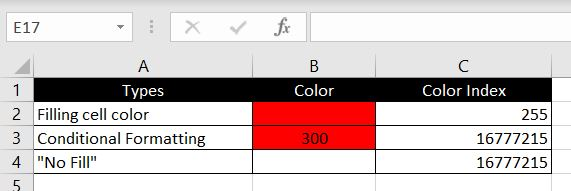As we can see, even with conditional formatting, the color index returned is the same for 2 & 3. From Excel’s perspective, a conditionally formatted cell has the same color index as a cell with no filled color. That is, provided of course the conditional formatted cell was not previously filled with a color.